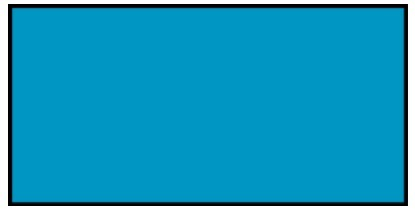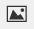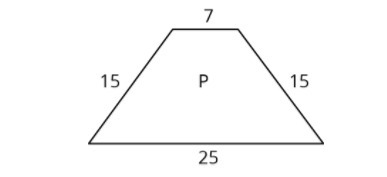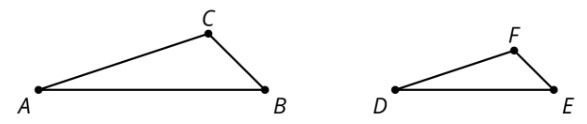PSA6QH3 - Practice Problems---7.1 Lesson 10: Changing Scales in Scale Drawings (7.G.A.1)
Part A)

Here is a scale drawing of a swimming pool where 1 cm represents 1 m.How long and how wide is the actual swimming pool?

Part B)

Will a scale drawing where 1 cm represents 2 m be larger or smaller than this drawing?

Select one:
Part C)

Make a scale drawing of the swimming pool where 1 cm represents 2 m.

Draw your drawing on paper, take a picture, and upload it using the image upload icon:If you do not have the ability to upload an image of your work type "Drawing is on paper."

Part A)

A map of a park has a scale of 1 inch to 1,000 feet. Another map of the same park has a scale of 1 inch to 500 feet. Which map is larger?

Select one:
Part B)

Part A)

On a map with a scale of 1 inch to 12 feet, the area of a restaurant is 60 in2. Han says that the actual area of the restaurant is 720 ft2. Do you agree or disagree?

Select one:
Part B)

If Quadrilateral Q is a scaled copy of Quadrilateral P created with a scale factor of 3, what is the perimeter of Q?Type your answer below as a number (example: 5, 3.1, 4 1/2, or 3/2):
Part A)

Triangle DEF is a scaled copy of triangle ABC. For each of the following parts of triangle ABC, identify the corresponding part of triangle DEF:

angle ABC?Select one:
Part B)

angle BCA?

Select one:
Part C)

segment AC?

Select one:
Part D)

segment BA?

Select one: